# 读取数据的格式

LI-6400 的数据格式要求很简单，表头不要修改，只保留导出 excel 或原始数据文件的表头和测量数据，使用你所掌握的任意方法将数据导入 R 软件即可，必须是 dataframe格式， LI-6800 的要求与 LI-6400 一致，样式分别如下：

## LI-6400 数据格式

Obs HHMMSS FTime EBal. Photo Cond Ci Trmmol VpdL CTleaf
1 11:17:23 247.5 0 17.8900448 1.2402490 363.8494 5.676993 0.6392769 30.79238
2 11:19:53 397.0 0 17.2727385 1.0476474 361.4220 5.257885 0.6678402 30.84335
3 11:22:23 547.5 0 16.8439480 0.8730468 357.2621 4.793988 0.6978914 30.88142
4 11:24:53 697.0 0 16.5556909 0.8113847 356.1285 4.563326 0.7029185 30.89113
5 11:27:23 847.5 0 15.2389528 0.6963616 353.1639 4.217108 0.7330305 30.95812
6 11:29:53 997.0 0 13.3778973 0.6098161 354.4347 3.912138 0.7575295 31.00215
7 11:32:23 1147.5 0 10.1900886 0.5499567 360.2758 3.513117 0.7415481 30.78868
8 11:34:53 1297.0 0 7.4913907 0.5044738 368.7882 3.358474 0.7623581 30.85906
9 11:37:23 1447.5 0 5.9274311 0.4705676 371.9833 3.538334 0.8516160 31.33811
10 11:39:53 1597.5 0 3.7266648 0.4339196 380.2271 3.290166 0.8492953 31.30909
11 11:42:24 1748.0 0 2.9387717 0.3849488 381.6673 2.974796 0.8527558 31.25271
12 11:44:53 1897.0 0 1.6870850 0.2977175 384.1619 2.755396 0.9931319 31.70684
13 11:47:22 2046.5 0 0.0214795 0.2463256 394.1682 2.341024 1.0034070 31.60617
14 11:49:54 2198.0 0 -1.3769278 0.2015835 405.3246 2.113570 1.0905067 31.90278

## LI-6800 数据格式

obs time elapsed date TIME E A Ca Ci Pci
1 1506482783 0.0 20170927 11:26:23 1506482783 0.0008851 10.3612425 386.135 171.6695 17.37742
2 1506482865 82.0 20170927 11:27:45 1506482865 0.0008621 10.3346065 386.287 174.8523 17.69907
3 1506482986 202.5 20170927 11:29:45 1506482985 0.0008013 9.9490963 386.775 176.6618 17.88154
4 1506483106 323.0 20170927 11:31:46 1506483106 0.0006955 8.9778485 388.149 176.2576 17.83885
5 1506483227 443.5 20170927 11:33:46 1506483226 0.0005794 7.5754675 389.944 178.2551 18.03889
6 1506483305 521.4 20170927 11:35:04 1506483304 0.0005022 6.0394698 391.955 199.7083 20.20909
7 1506483425 641.9 20170927 11:37:05 1506483425 0.0004196 4.9187258 393.488 207.6760 21.01452
8 1506483510 726.4 20170927 11:38:29 1506483509 0.0003747 4.0239474 394.701 225.4976 22.81878
9 1506483595 811.4 20170927 11:39:54 1506483594 0.0003379 2.7471387 396.215 266.6744 26.98560
10 1506483676 892.4 20170927 11:41:15 1506483675 0.0003094 2.0662518 397.195 289.2526 29.26888
11 1506483749 965.4 20170927 11:42:28 1506483748 0.0002783 0.8054445 398.814 349.0914 35.32387
12 1506483820 1036.4 20170927 11:43:39 1506483819 0.0002683 -0.0551727 399.882 396.9526 40.16528
13 1506483906 1122.4 20170927 11:45:05 1506483905 0.0002566 -0.8092610 400.904 442.0830 44.73083

# 使用方法

devtools::install_github("zhujiedong/lrc_start")
library(lrc.start)

• 直角双曲线模型
• plot_nonrec64: 适用于 LI-6400 数据的模型
• plot_nonrec68: 适用于 LI-6400 数据的模型
• 非直角双曲线模型
• plot_rec64: 适用于 LI-6400 数据的模型
• plot_rec68: 适用于 LI-6400 数据的模型
• 指数模型
• plot_exp64: 适用于 LI-6400 数据的模型
• plot_exp68: 适用于 LI-6400 数据的模型

lrc.start 的实现方法很简单，首先构造初始值的一系列梯度，受限于我的水平，目前直角双曲线和非直角双曲线都是构建 “alpha” 的梯度，例如，假设 alpha 最小值是 0.01，所有的测量值为 13 个，那么 alpha 的变化范围是 0.01 到 0.01 * 13， 以 0.01 为间隔增长，指数模型改变的梯度为 b，变化方式同 alpha，其他数值， Am 以数据中最大的光合速率加最小光合速率的绝对值（也即光强为 0 时的测量值）作为初始值。Rd 以最小光合速率的绝对值作为初始值。具体请看下面的内容。

## 直角双曲线模型

Baly (1935) 提出了直角双曲线模型，它的表达式为：

$P_{n} = \frac{\alpha I\ P_{nmax}}{\alpha I + P_{nmax}}- R_{d}$

• 其中，P$$_{n}$$ 为净光合速率；
• 为光强；
• $$\alpha$$ 为光响应曲线在光强为0时的斜率，即光响应曲线的初始斜率，也称之为初始量子效率；
• P$$_{nmax}$$ 为最大净光合速率，在函数参数中我们使用 Am 表示；
• R$$_{d}$$：为暗呼吸速率。

### 实现方式

# start64 为我测试时的数据，已经内置到lrc.start 内
plot_rec64(start64)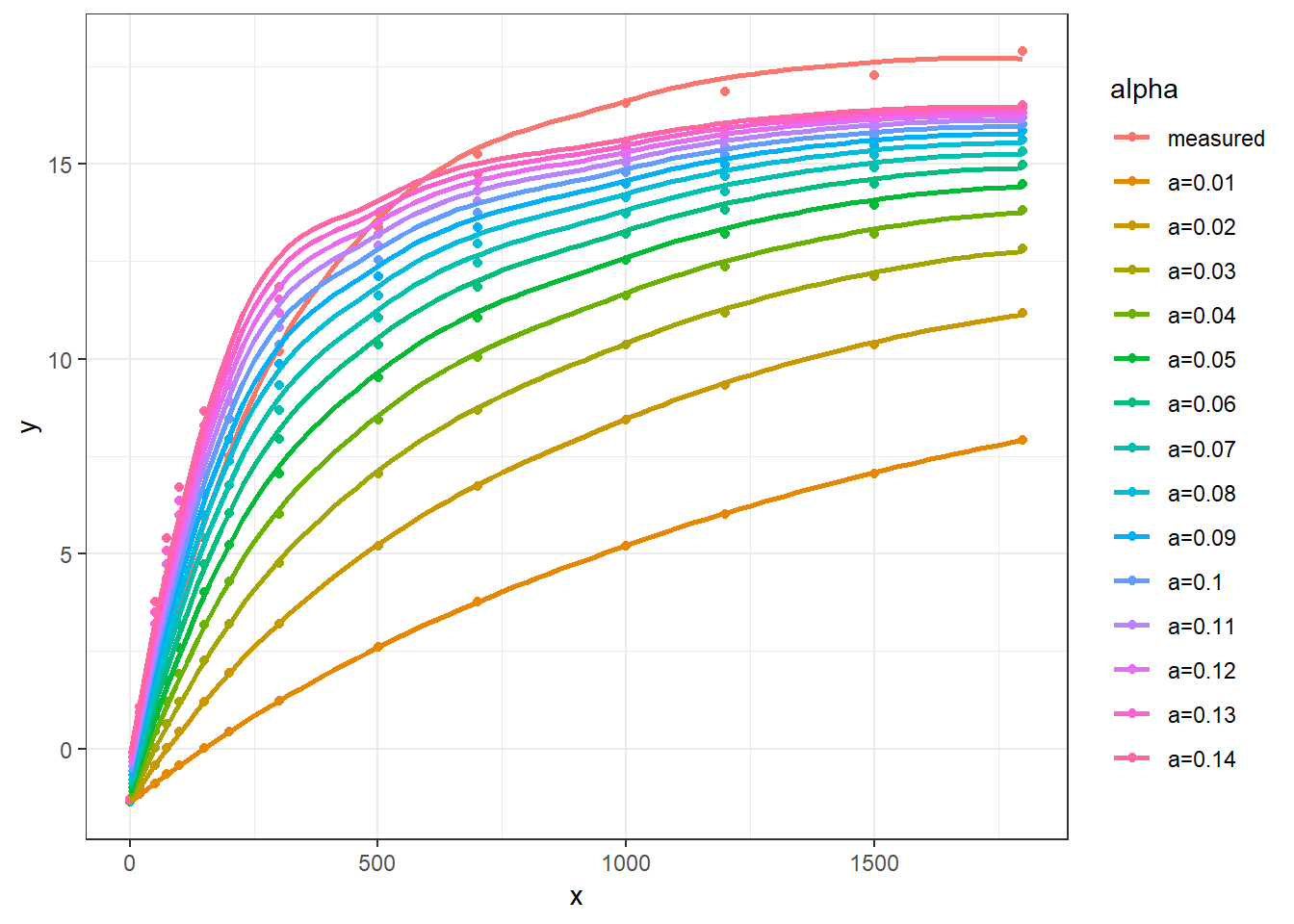plot_rec64(start64, Am = 25)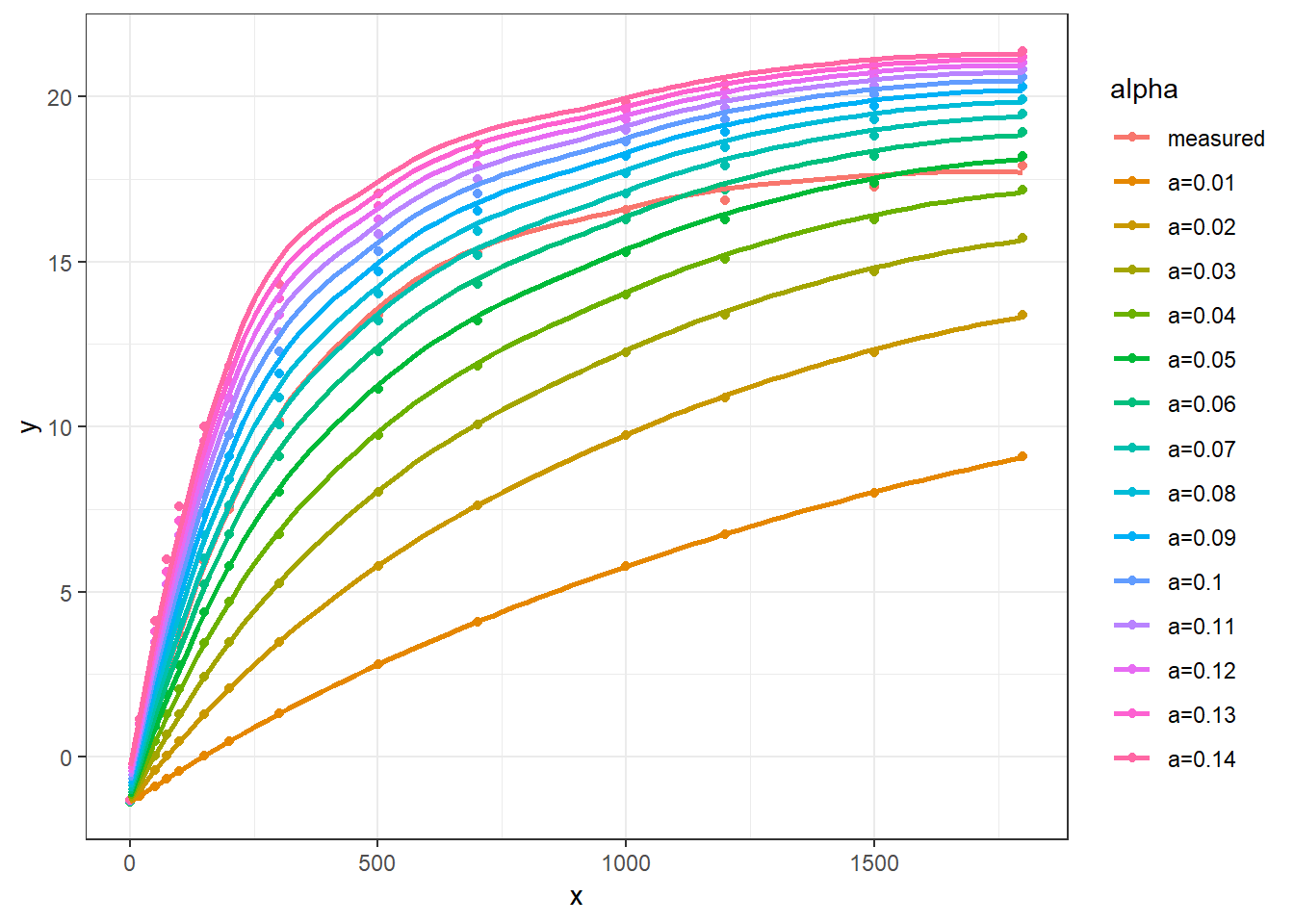lrc_Q <- start64$PARi lrc_A <- start64$Photo

lrcnls <- nls(lrc_A ~ (alpha * lrc_Q * Am) *
(1/(alpha * lrc_Q + Am)) - Rd,
start=list(Am=(max(lrc_A)-min(lrc_A)),
alpha=0.05,Rd=-min(lrc_A))
)
summary(lrcnls)
##
## Formula: lrc_A ~ (alpha * lrc_Q * Am) * (1/(alpha * lrc_Q + Am)) - Rd
##
## Parameters:
##        Estimate Std. Error t value Pr(>|t|)
## Am    23.124056   0.357404  64.700 1.49e-15 ***
## alpha  0.078754   0.004846  16.252 4.89e-09 ***
## Rd     1.647967   0.256492   6.425 4.91e-05 ***
## ---
## Signif. codes:  0 '***' 0.001 '**' 0.01 '*' 0.05 '.' 0.1 ' ' 1
##
## Residual standard error: 0.3589 on 11 degrees of freedom
##
## Number of iterations to convergence: 5
## Achieved convergence tolerance: 1.462e-06

## 非直角双曲线模型

Thornley (1976) 提出了非直角双曲线模型，它的表达式为：

$P_{n} = \frac{\alpha I + P_{nmax} \sqrt{(\alpha I + P_{nmax})^{2} - 4 \theta \alpha I P_{nmax}}}{2 \theta} - R_{d}$

### 实现方式

library(lrc.start)
plot_nonrec68(start68)
## geom_smooth() using method = 'loess' and formula 'y ~ x'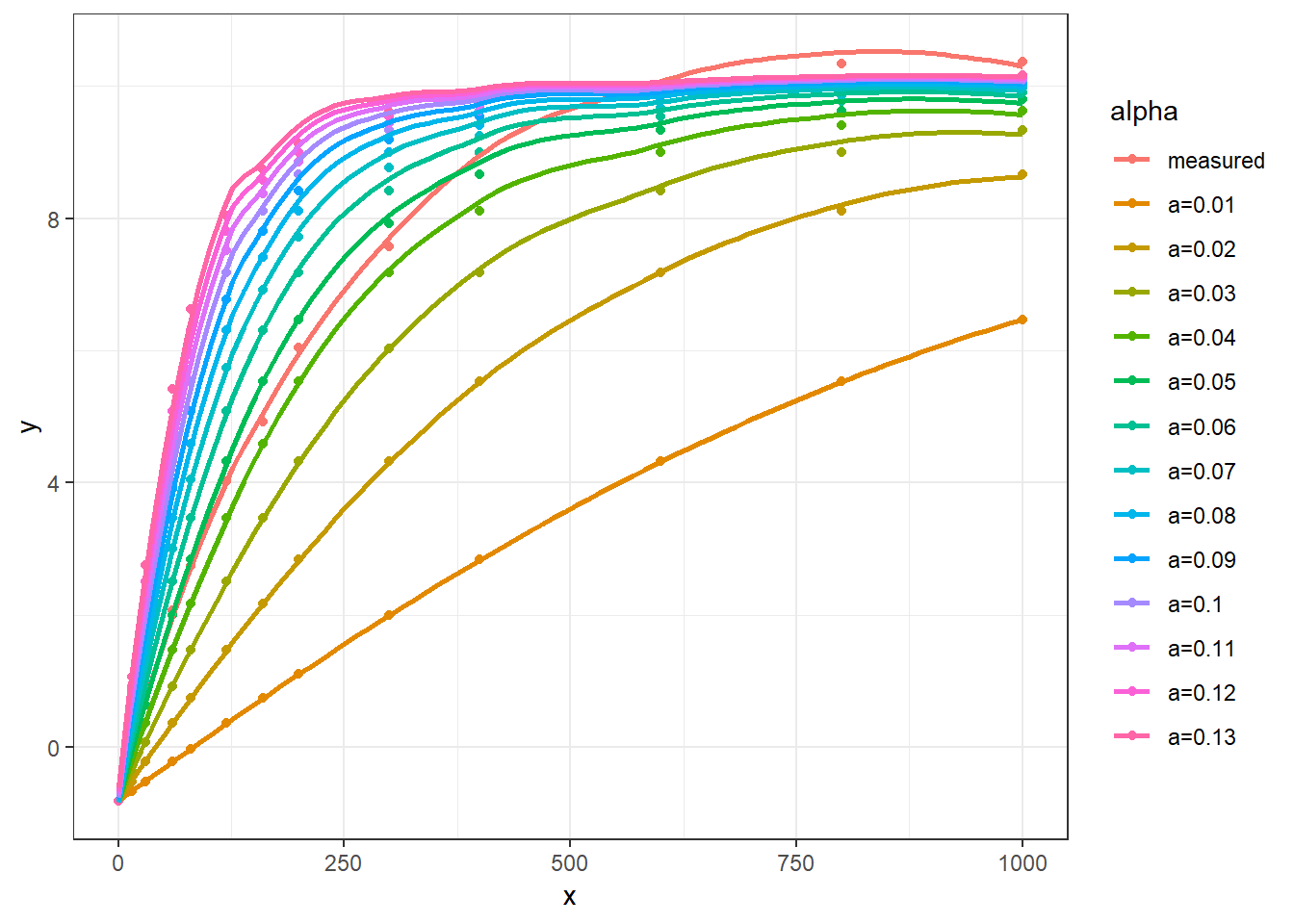library(lrc.start)
plot_nonrec68(start68, Am = 12)
## geom_smooth() using method = 'loess' and formula 'y ~ x'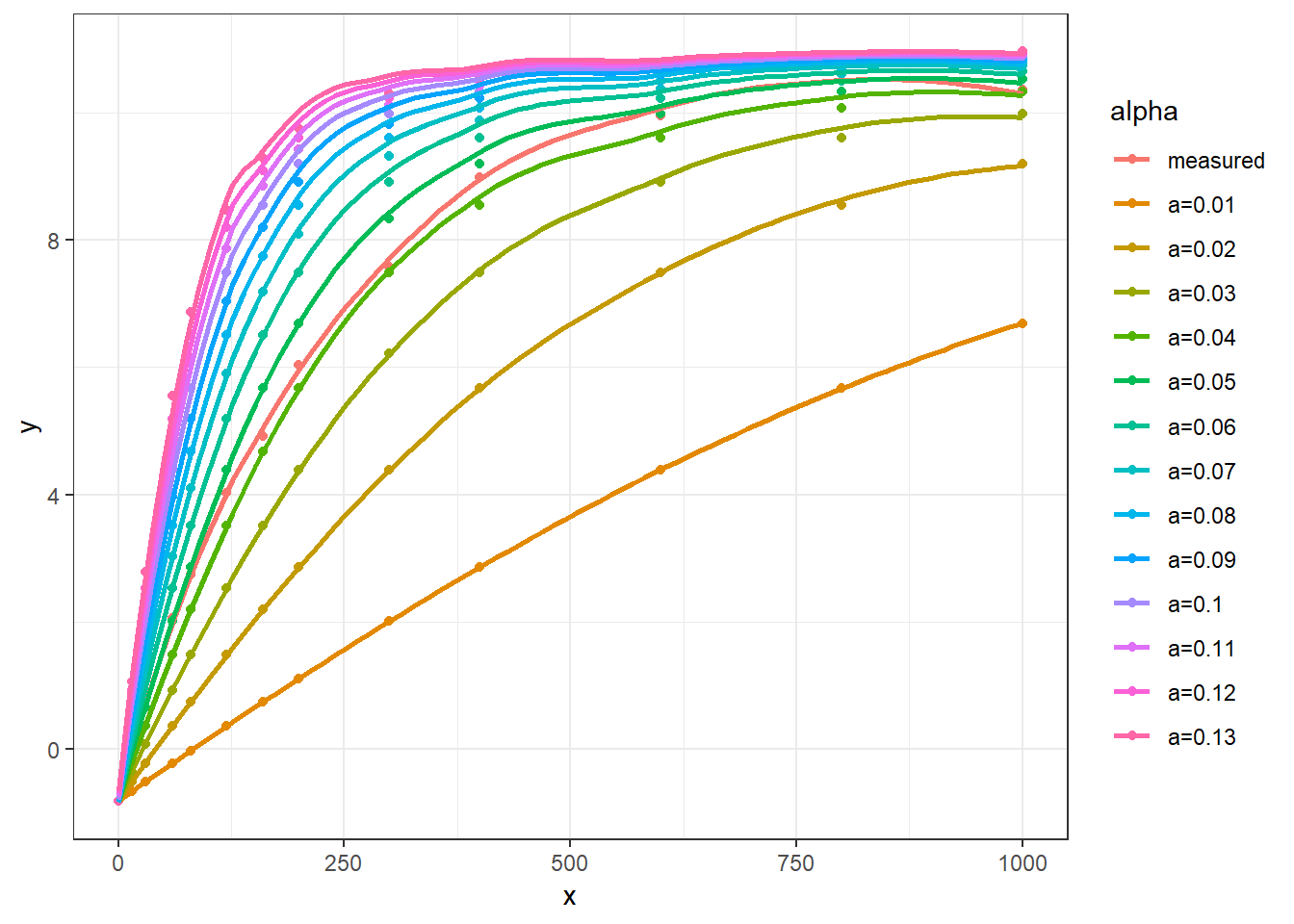## 指数模型

$P_{n} = P_{nmax}[1 - e^{-b(I-I_{C})}]$

### 实现方式

library(lrc.start)
plot_exp64(start64)
## geom_smooth() using method = 'loess' and formula 'y ~ x'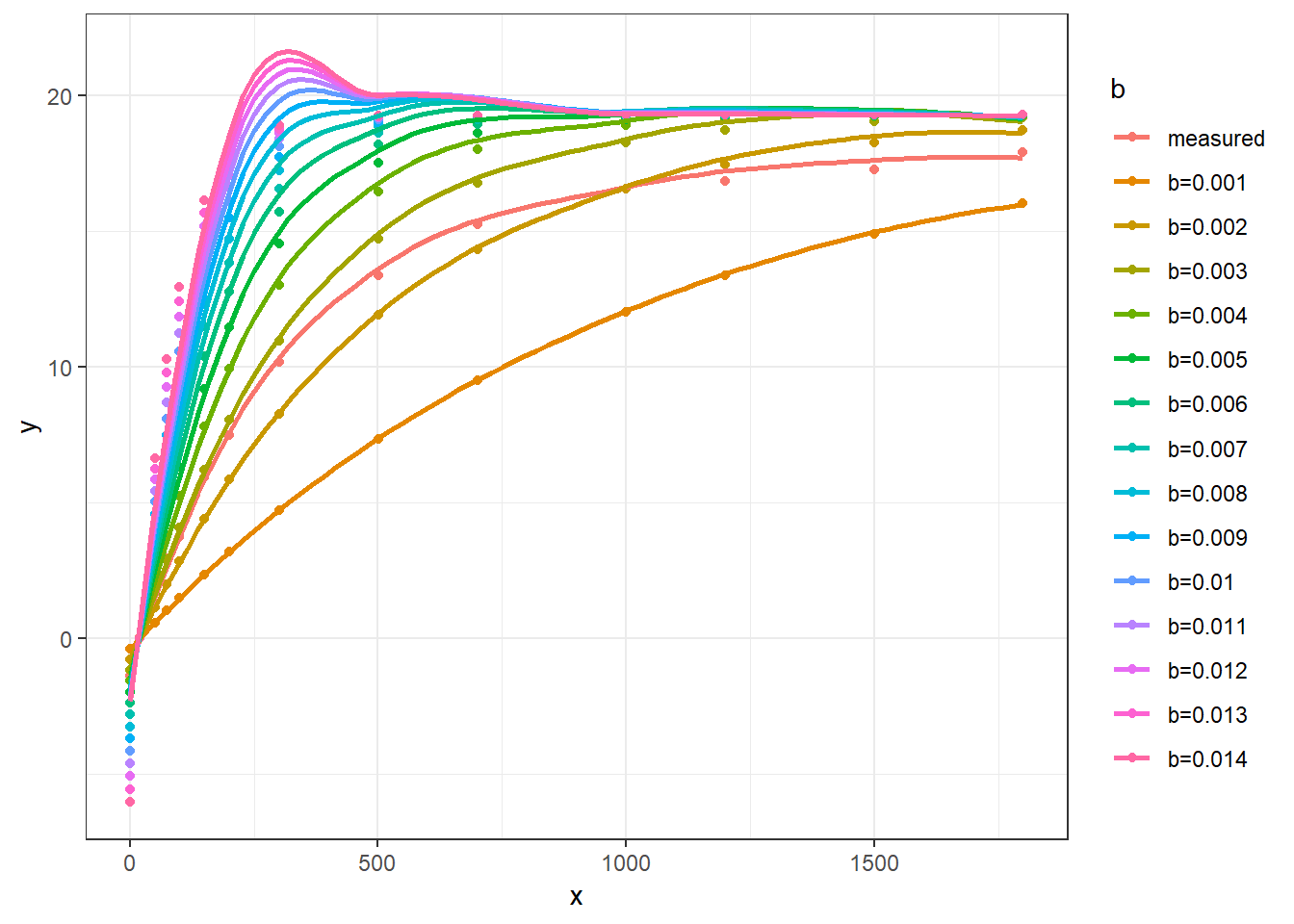library(lrc.start)
plot_exp64(start64, Am = 18)
## geom_smooth() using method = 'loess' and formula 'y ~ x'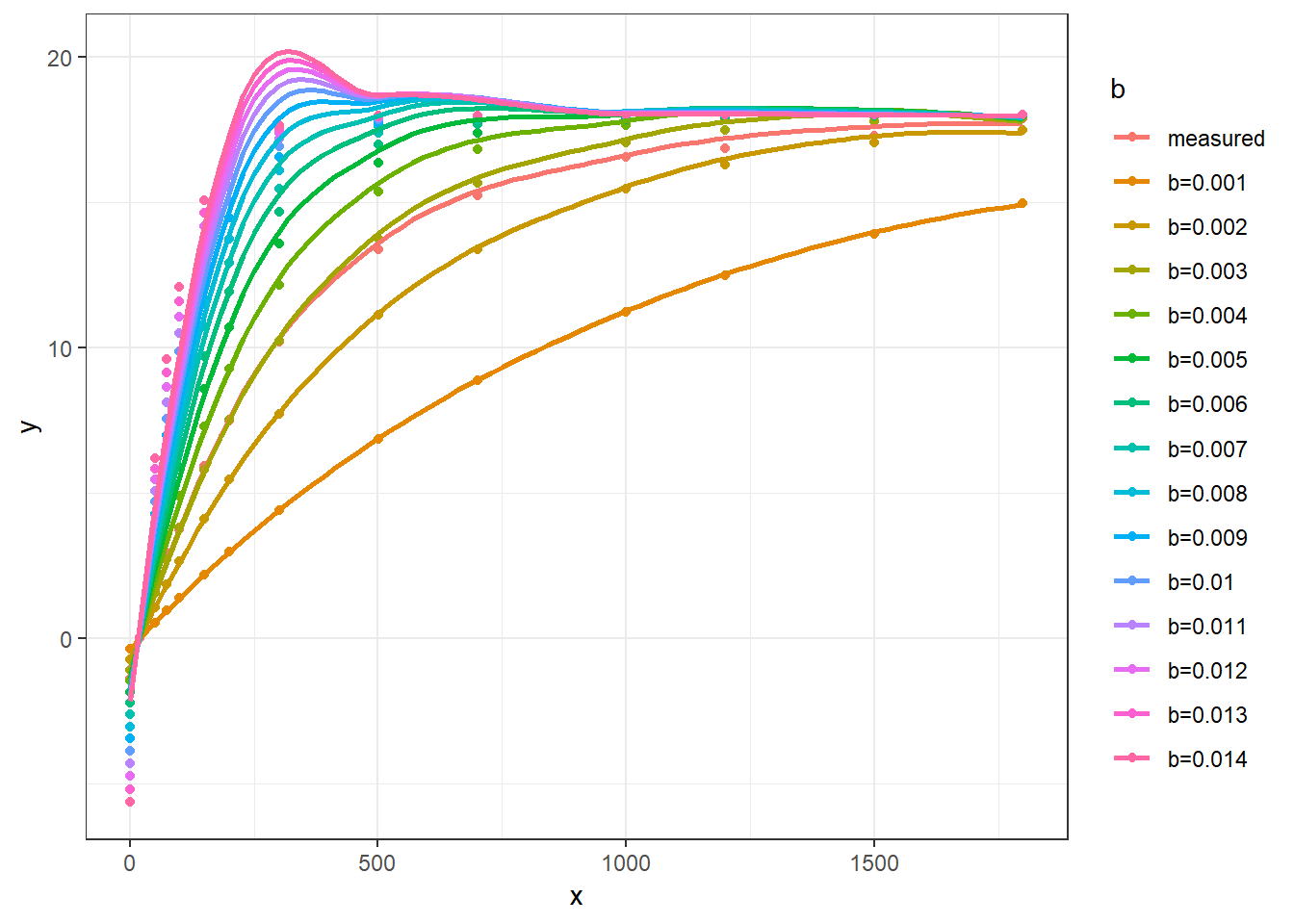lrc_Q <- start64$PARi lrc_A <- start64$Photo

lrcnls <- nls(lrc_A ~ Am*(1-exp((-b)*(lrc_Q-Ic))),
start=list(Am=18,
Ic=20, b=0.003)
)
summary(lrcnls)
##
## Formula: lrc_A ~ Am * (1 - exp((-b) * (lrc_Q - Ic)))
##
## Parameters:
##     Estimate Std. Error t value Pr(>|t|)
## Am 1.751e+01  1.241e-01 141.154  < 2e-16 ***
## Ic 1.982e+01  2.031e+00   9.761 9.41e-07 ***
## b  3.081e-03  7.833e-05  39.338 3.47e-13 ***
## ---
## Signif. codes:  0 '***' 0.001 '**' 0.01 '*' 0.05 '.' 0.1 ' ' 1
##
## Residual standard error: 0.2122 on 11 degrees of freedom
##
## Number of iterations to convergence: 4
## Achieved convergence tolerance: 2.302e-06

# 参考文献

Baly E. 1935. The kinetics of photosynthesis. Proceedings of the Royal Society of London Series B (Biological Sciences), 218–239.

Prado CH, Moraes JAPVD. 1997. Photosynthetic capacity and specific leaf mass in twenty woody species of cerrado vegetation under field conditions. Photosynthetica 33, 103–112.

Thornley JHM. 1976. Mathematical models in plant physiology. London: Academic Press.

...

... 本站访问量：   您是本站第 位访问者